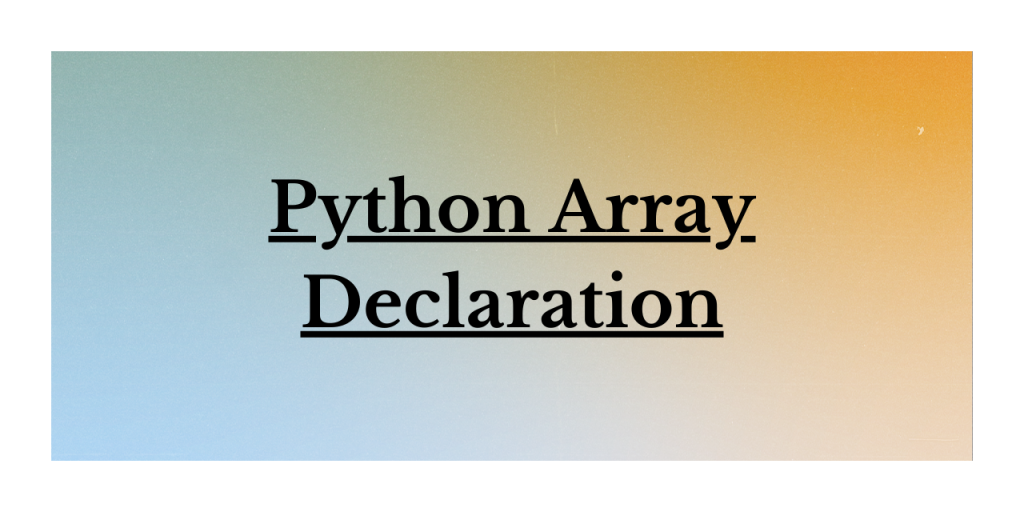# Python Array Declaration: A Comprehensive Guide for BeginnersIn this article, we discuss different methods for declaring an array in Python, including using the Python Array Module, Python List as an Array, and Python NumPy Array. We also provide examples and syntax for each method, as well as a brief overview of built-in methods for working with arrays in Python. Let’s get started!

## What is a Python Array?

As we all know, Python offers various data structures to manipulate and deal with the data values.

When it comes to ARRAY as a data structure, Python does not offer a direct way to create or work with arrays. Rather, it provides us with the below variants of Array:

• Python Array Module: The Array module contains various methods to create and work with the values.
• Python List: List can be considered as a dynamic array. Moreover, heterogeneous elements can be stored in Lists, unlike Arrays.
• Python NumPy Array: NumPy arrays are best suitable for mathematical operations to be performed on a huge amount of data.

Having understood Python Arrays, let us now understand the ways through which we can declare an array in Python.

## Python Array Declaration: Different Variants

The Python Array Module provides an array data structure that can store elements of the same type, such as integers or characters. It is more efficient than lists when it comes to memory usage, especially for large datasets. In this method, we use the array() function from the array module to create an array in Python.

In Python, you can declare arrays using the Python Array Module, Python List as an Array, or Python NumPy Array. The Python Array Module and NumPy Array offer more efficient memory usage and specific data types, while Python lists provide flexibility with dynamic sizing and heterogeneous elements. Built-in methods are available to manipulate and manage data within these arrays.

### Array Declaration Using Python Array Module

`Python Array module `contains` array() function`, using which we can create an array in the python environment.

Syntax:

```array.array('format code',[data])
```
• `format_code`: It represents the type of elements to be accepted by an array. The code ‘i’ represents numeric values, while ‘f’ represents floating-point numbers.

Example:

```import array
arr = array.array('i', [10,20,30,40,50])
print(arr)
```

Output:

```array('i', [10, 20, 30, 40, 50])
```

We’ve imported the array module and created an array `arr` using the `array()` function with the format code ‘i’ (indicating that the array should store signed integers). We then passed a list of integers `[10, 20, 30, 40, 50]` as the data for the array. At the end, we printed the array, to show it with the specified format code and elements.

### Array Declaration Using Python List as an Array

Python lists are versatile and can be used as a more flexible alternative to arrays. Lists store elements of different data types, change their size dynamically, and offer a variety of built-in methods for manipulating the data. In this method, we use the Python list to store and manage elements like an array.

Syntax:

```list = [data]
```

Example:

```lst = [10,20,30,40, 'Python']
print(lst)
```

Output:

```[10, 20, 30, 40, 'Python']
```

In this example, we’re working with Python lists as an array. Here, we’ve created a variable named lst which contains both integers and a string named “Python”. Because lists do not care about the data that you enter in them, list arrays are quite flexible compared to the Python array module.

### Array Declaration Using Python NumPy Array

The `NumPy module` contains various functions to create and work with array as a data structure. The `numpy.array() function` can be used to create single as well as multi-dimensional array in Python. It creates an array object as ‘ndarray’.

```np.array([data])
```

Example: Array creation using numpy.array() function

```import numpy
arr = numpy.array([10,20])
print(arr)
```

Output:

```[10 20]
```

Further, we can use `numpy.arange() function` to create an array within the specific range of data values.

```numpy.arange(start,stop,step)
```
• `start`: The starting element of the array.
• `end`: The last element of the array.
• `step`: The number of interval or steps between array elements.

Example:

```import numpy
arr = numpy.arange(1,10,2)
print(arr)
```

Output:

```[1 3 5 7 9]
```

## Methods of An Array

Python has a set of built-in methods that you can use on lists/arrays.

## Summary

In this article, we’ve covered various methods to declare and work with arrays in Python, such as the Python Array Module, Python List as an Array, and Python NumPy Array. We also discussed built-in methods that can be used on lists and arrays. Understanding these concepts will help you effectively manage and manipulate data in your Python projects. If you have any questions or suggestions, feel free to leave a comment below.